# GMAT Math : DSQ: Understanding fractions

## Example Questions

### Example Question #1 : Fractions

Of the marbles in a box,are blue,are red, and the rest are green. Also, half of the marbles are large and half are small. How many marbles are there?

1) Half of the blue marbles and half of the red marbles are large.

2) There are thirty-six large marbles that are either red or green.

BOTH statements TOGETHER are not sufficient to answer the question.
Statement 2 ALONE is sufficient to answer the question, but Statement 1 ALONE is not sufficient to answer the question.
Statement 1 ALONE is sufficient to answer the question, but Statement 2 ALONE is not sufficient to answer the question.
BOTH statements TOGETHER are sufficient to answer the question, but NEITHER statement ALONE is sufficient to answer the question.
EITHER statement ALONE is sufficient to answer the question.
Correct answer: BOTH statements TOGETHER are sufficient to answer the question, but NEITHER statement ALONE is sufficient to answer the question.
Explanation:

From the initial information, we can determine that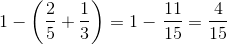of the balls are green.

The first statement alone tells you that half of the blue marbles and half of the red marbles are large, so half of the green marbles must be large as well. But this alone does not tell you how many marbles there are total.

The second statement alone tells you how many large marbles are red or green, but you do not have any way of figuring out how many small marbles or total marbles are.

But if you put the statements together, you know the following:

Half of the red marbles and half of the green marbles comprise the thirty-six large marbles: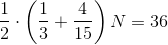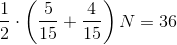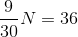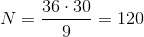There are 120 marbles.

The answer is that both statements together are sufficent to answer the question, but neither statement alone is sufficient.

### Example Question #2 : Fractions

Is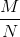a  fraction in lowest terms?

Statement 1:is a multiple of 4 greater than 8.

Statement 2:is an even number greater than 6.

BOTH statements TOGETHER are sufficient to answer the question, but NEITHER statement ALONE is sufficient to answer the question.

BOTH statements TOGETHER are insufficient to answer the question.

EITHER statement ALONE is sufficient to answer the question.

Statement 2 ALONE is sufficient to answer the question, but Statement 1 ALONE is NOT sufficient to answer the question.

Statement 1 ALONE is sufficient to answer the question, but Statement 2 ALONE is NOT sufficient to answer the question.

BOTH statements TOGETHER are sufficient to answer the question, but NEITHER statement ALONE is sufficient to answer the question.

Explanation:

Both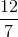and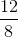satisfy the conditions of Statement 1; the first fraction is in lowest terms, the second is not.

Both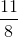andsatisfy the conditions of Statement 2; the first fraction is in lowest terms, the second is not.

Neither statement alone is enough to answer the question. Both together, however, are enough to prove the fraction is not in lowest terms; a fraction which satisfies both statements has both its numerator and denominator divisible by 2.

### Example Question #3 : Fractions

Is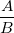a  fraction in lowest terms?

Statement 1:is an odd number divisible by 5.

Statement 2:is an even number not divisible by 5.

BOTH statements TOGETHER are insufficient to answer the question.

Statement 2 ALONE is sufficient to answer the question, but Statement 1 ALONE is NOT sufficient to answer the question.

EITHER statement ALONE is sufficient to answer the question.

Statement 1 ALONE is sufficient to answer the question, but Statement 2 ALONE is NOT sufficient to answer the question.

BOTH statements TOGETHER are sufficient to answer the question, but NEITHER statement ALONE is sufficient to answer the question.

BOTH statements TOGETHER are insufficient to answer the question.

Explanation:

The two statements together are insufficient.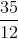and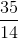each satisfy the conditions of both statements, but the first fraction is in lowest terms and the second is not.

### Example Question #4 : Fractionsis a fraction in lowest terms. Is its decimal representation a terminating decimal or a repeating decimal?

Statement 1:is a power of 2.

Statement 2:is a multiple of 5.

EITHER statement ALONE is sufficient to answer the question.

Statement 1 ALONE is sufficient to answer the question, but Statement 2 ALONE is NOT sufficient to answer the question.

Statement 2 ALONE is sufficient to answer the question, but Statement 1 ALONE is NOT sufficient to answer the question.

BOTH statements TOGETHER are insufficient to answer the question.

BOTH statements TOGETHER are sufficient to answer the question, but NEITHER statement ALONE is sufficient to answer the question.

BOTH statements TOGETHER are insufficient to answer the question.

Explanation:

The two statements together provide insufficient information.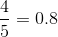and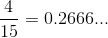are two examples of fractions that satisfy both conditions; note that the first is represented in decimal form by a terminating decimal, and the second, by a repeating decimal.

### Example Question #5 : Fractions

Which fraction is larger than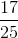?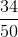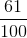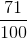Explanation:

From the question, we know that only one of the fractions in the answer choices is larger than. The correct answer must therefore be the largest of the answer choices. This observation is important, because it means we can eliminate some of the answer choices without performing any calculations. For example,is smaller than, so eliminatefrom the possibilities.is smaller than bothand, so eliminate it as well. Similarly,is smaller than, so eliminatetoo.

This leaves us with only two answer choices:andFirst consider. We can rewrite this as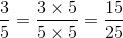, which is clearly smaller than. Eliminate.

By process of elimination, we've thus shown thatis the correct answer choice.

### Example Question #6 : Fractionsis a real number. True or false:is an integer.

Statement 1: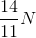is an integer.

Statement 2: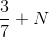is an integer.

BOTH STATEMENTS TOGETHER provide sufficient information to answer the question, but NEITHER STATEMENT ALONE provides sufficient information to answer the question.

BOTH STATEMENTS TOGETHER do NOT provide sufficient information to answer the question.

STATEMENT 2 ALONE provides sufficient information to answer the question, but STATEMENT 1 ALONE does NOT provide sufficient information to answer the question.

STATEMENT 1 ALONE provides sufficient information to answer the question, but STATEMENT 2 ALONE does NOT provide sufficient information to answer the question.

EITHER STATEMENT ALONE provides sufficient information to answer the question.

STATEMENT 2 ALONE provides sufficient information to answer the question, but STATEMENT 1 ALONE does NOT provide sufficient information to answer the question.

Explanation:

Statement 1 alone does not proveis or is not an integer.

For example, if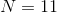, then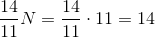.

If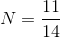, then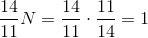.is an integer in only one scenario, but Statement 1 is true in both.

Now assume Statement 2 alone, and let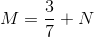. Thenis an integer by Statement 2, and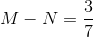Supposeis an integer. Then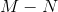, the difference of integers, is itself an integer. But we know that this is equal to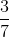, which is not an integer, so, by contradiction,is not an integer.